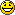# the peculiar number 2π-6##the peculiar number 2π-6

From this number i derived the following class of functions

z=F(x,y)/(1-N/(N+F(x,y))) where in this case N=3 and F(x,y)=x*y with y set to 1.

This i find can be relaxed to any functions F(),G() and and any integers M,N

z=F(x,y)/(1-M/(N+G(x,y))).

My particular favourite is
z=sin(x)/(1-3/(2+sin(y))).

Hope you enjoy playing with thisjehovaja
GuestPermissions in this forum:
You cannot reply to topics in this forum